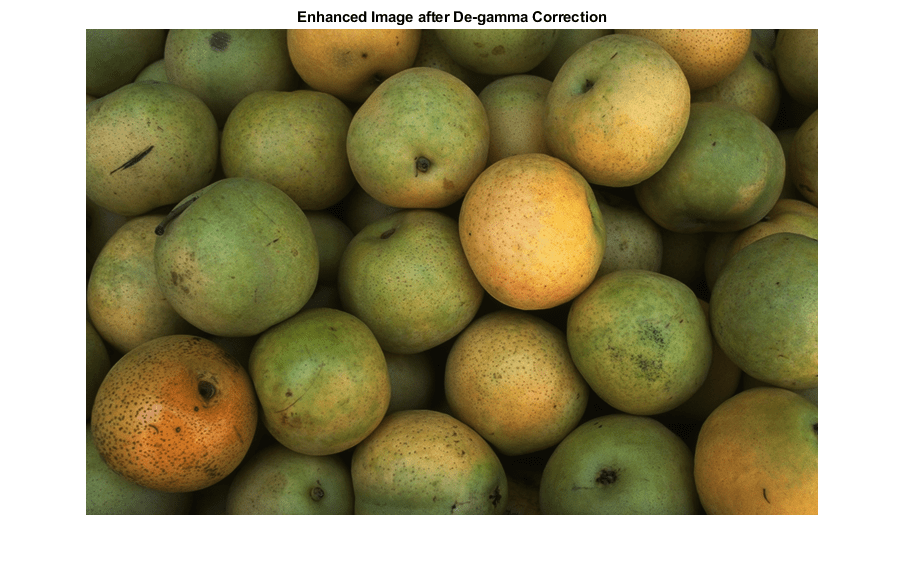Main Content

# vision.GammaCorrector

Apply or remove gamma correction from images or video streams

## Description

To apply gamma correction to input images or a video stream:

1. Create the `vision.GammaCorrector` object and set its properties.

2. Call the object with arguments, as if it were a function.

To learn more about how System objects work, see What Are System Objects?.

## Creation

### Syntax

``gammaCorr = vision.GammaCorrector``
``gammaCorr = vision.GammaCorrector(Name,Value)``
``gammaCorr = vision.GammaCorrector(gamma,Name,Value)``

### Description

example

````gammaCorr = vision.GammaCorrector` returns a gamma corrector object, `gammaCorr`. You can use the gamma corrector to apply or remove gamma correction from images or video streams.```
````gammaCorr = vision.GammaCorrector(Name,Value)` sets properties using one or more name-value pairs. Enclose each property name in quotes. For example, ```gammaCorr = vision.GammaCorrector('Correction','Gamma')``````
````gammaCorr = vision.GammaCorrector(gamma,Name,Value)` additionally sets the `Gamma` property.```

## Properties

expand all

Unless otherwise indicated, properties are nontunable, which means you cannot change their values after calling the object. Objects lock when you call them, and the `release` function unlocks them.

If a property is tunable, you can change its value at any time.

For more information on changing property values, see System Design in MATLAB Using System Objects.

Specify gamma correction or linearization, specified as `'Gamma'` or `'De-gamma'`.

Gamma value of output or input, specified as numeric scalar greater than or equal to `1`. When you set the `Correction` property to `Gamma`, this property gives the desired gamma value of the output video stream. When you set the `Correction` property to `De-gamma`, this property indicates the gamma value of the input video stream.

Enable gamma curve to have linear portion near origin, specified as `true` or `false`.

I-axis value of the end of gamma correction linear segment, specified as a numeric scalar value in the range (0,1). This property applies when you set the `LinearSegment` property to `true`.

## Usage

### Syntax

``y = gammaCorr(input)``

### Description

example

````y = gammaCorr(input)` applies or removes gamma correction from input `I`, and returns the gamma corrected or linearized output `y`.```

### Input Arguments

expand all

Input, specified as an M-by-N matrix of intensity values or M-by-N-P color video, where P is the number of color planes.

### Output Arguments

expand all

Output, returned as an M-by-N matrix of intensity values or M-by-N-P color video, where P is the number of color planes.

## Object Functions

To use an object function, specify the System object™ as the first input argument. For example, to release system resources of a System object named `obj`, use this syntax:

`release(obj)`

expand all

 `step` Run System object algorithm `release` Release resources and allow changes to System object property values and input characteristics `reset` Reset internal states of System object

## Examples

collapse all

Create a gamma corrector object with the gamma property set to `2.0.`

`hgamma = vision.GammaCorrector(2.0,'Correction','De-gamma');`

Read an image.

`img = imread('pears.png');`

Apply gamma correction.

`imgCor = hgamma(img);`

Show the original and corrected images.

`imshow(img); title('Original Image');````figure, imshow(imgCor); title('Enhanced Image after De-gamma Correction');```## See Also

Introduced in R2012a

Download ebook